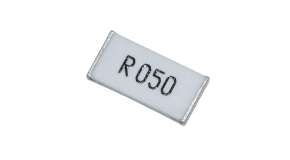# 0805 | 0402 | 2512贴片电阻参数介绍

SMD贴片电阻器也是一种卡式固定电阻器，是金属玻璃釉电阻器之一。0805、0402和2512是贴片电阻器中常用的贴片电阻器封装形式。0805、0402和2512的电阻值分别是多少？很多时候，作为一个企业，我们面临着许多根本不存在的阻力值，然后我们必须对它们进行筛选。关键问题是没有阻力表可供应用。贴片电阻根据e24-e96系列确定。电阻力
电阻用来表示导体对电流的电阻。导体对电流的电阻越大所以电阻就越大。不同导体的电阻通常不同。导体本身的特性也就是电阻。电阻元件是阻碍电流的耗能元件。电阻由电阻值表示。
电阻值的换算关系
贴片电阻值的误差精度为±1％，±2％，±5％，±10％。最常用的是±1％和±5％，±5％的准确度通常用三位数字表示。电阻值的计算如下：
Ω= Ω
k=kΩ=1000Ω
M=MΩ=1000000Ω
0805 | 0402 | 2512贴片电阻计
0805电阻0402电阻2512电阻
1R1=0.1Ω270=27Ω392=3.9KΩ
R22=0.22Ω330=33Ω472=4.7KΩ
R33=0,33Ω390=39Ω562=5.6KΩ
R47=0.47Ω470=47Ω682=6.8KΩ
R68=0.68Ω560=56Ω822=8.2KΩ
R82=0.82Ω680=68Ω103=10KΩ
1R0=1Ω820=82Ω123=12KΩ
1R2=1.2Ω101=100Ω153=15KΩ
2R2=2.2Ω181=180Ω183=18KΩ
3R3=3.3Ω221=220Ω223=22KΩ

2022-05-20
2022-05-20
2022-05-20
2022-05-19
2022-05-19
2022-05-19
2022-05-18
2022-05-18
2022-05-18
2022-05-17
2022-05-17
2022-05-16